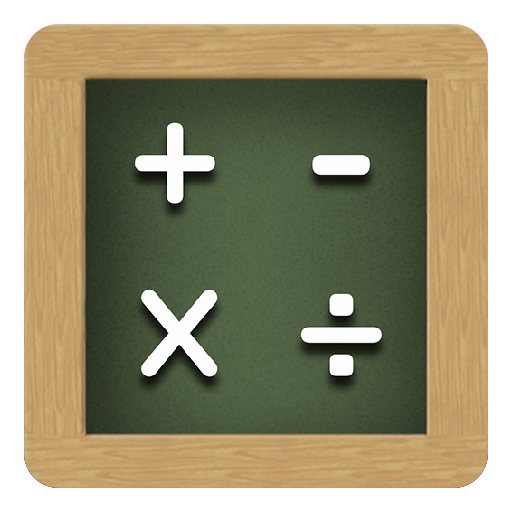## Crazy Maths

### by Movilfin

Free
2062
Categories Tags
###### Description

Exploring a variety of mathematical topics.
Learn basic math skills in addition, subtraction, multiplication and division.

Learn the even numbers, odd numbers, prime numbers, Fibonacci numbers.
Learn multiplication table.
Exploring math skills.

Improves arithmetic fluency and promotes development of mental strategies for addition, subtraction, multiplication, division and mixed operations. With a focus on self-improvement.

FEATURES / EDUCATIONAL CONTENT:
- Subtraction
- Multiplication
- Division
- Arithmetic
- Multiplication table
- The even numbers, odd numbers, prime numbers, Fibonacci numbers.
- Combination of the above
- Practice mental addition, subtraction, multiplication, division and mixed operations
- Develop arithmetic fluency and improve mental strategies
- Mental math game
- Choose their options by themselves
- Various fun game modes
- Possibility to follow the results of several players
- 3 levels of difficulty
- Fun animations
- High scores and awards.

We are listening to, frequently enhancements game based on your feedback
Please give us feedback in comments, we will make it the best game!

1177
• GOTD

490